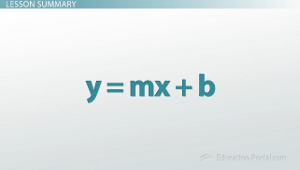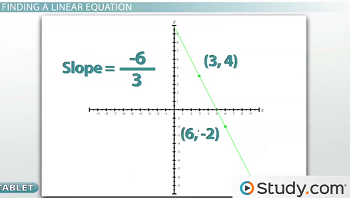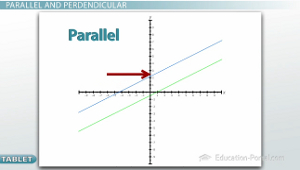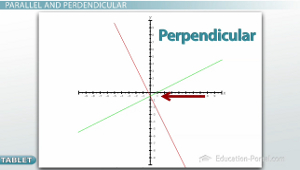# How to Write a Linear Equation

An error occurred trying to load this video.

Try refreshing the page, or contact customer support.

Coming up next: What is a System of Equations?

### You're on a roll. Keep up the good work!

Replay
Your next lesson will play in 10 seconds
• 0:05 Finding a Linear Equation
• 3:19 Parallel and Perpendicular
• 5:01 Finding Parallel Equations
• 6:32 Solving Perpendicular…
• 8:04 Lesson Summary

Want to watch this again later?

Timeline
Autoplay
Autoplay
Speed

#### Recommended Lessons and Courses for You

Lesson Transcript
Instructor: Jeff Calareso

Jeff teaches high school English, math and other subjects. He has a master's degree in writing and literature.

Simply knowing how to take a linear equation and graph it is only half of the battle. You should also be able to come up with the equation if you're given the right information.

## A Mathematician's NeedsMathematicians like to be thorough and find all the connections between things. So just being able to go from an algebraic rule to drawing the graph isn't really good enough for them. They want to be able to go the other way, too. Going from the graph to the rule isn't too bad because you can just pick out the information you need. But sometimes the picture isn't always going to be given and only small pieces of information will be given to you, and it's up to you to fill in the gaps.

## Finding a Linear Equation

There's a lot of different kinds of information that could be given. The most common is that you'll be given two points. Maybe they'll ask you to find the equation of the line between the points (3,4) and (6,-2).

For any linear equation, the two things we need to know are the slope and the y-intercept. The slope is every time going to be the first thing we should try to find. Once we know the slope, it's a lot easier to find the y-intercept.

I personally like to find the slope when I'm given two points just using the logic that I know because I know the slope is the rise over the run, which means how much it goes up and down over how much it goes left and right. I know the rise is the change in y. So in this one, I see the y starting at 4 and going down to -2, which means the rise is -6. The run, the left and right, is x, and I see it start at 3 and go up to 6, which is a change of 3. And so I can get the slope right from this, which is -2.A lot of people like using the formula, which is the x1, y1, x2, y2 formula. If you do it this way, you'll get the exact same answer. You plug in numbers y2 minus y1 over x2 minus x1 (y2 - y1 / x2 - x1). You do -2 -and get -6. And 6 - 3 = 3. And again, we end up with a slope of -2.

Like I said earlier, the two things we need are the slope and the y-intercept. We now know what the slope is, which means the y-intercept is the only thing left to find. Because I know m (-2) and because it gave us a sample x and y (3,4), I can substitute in everything I know and I'm left with an equation with only one variable in it (4 = -2 * 3 + b).

First, I do the operation it asks us to: -2 * 3 = -6. Then, I have to get the b by itself, which means I undo the -6. I undo subtraction with addition because of inverse operations. I end up with b = 10. Now that I know m and I know b, I'm done, and my answer is y = -2x + 10. That means that this linear equation begins at 10 and is decreasing by 2 every step of the way.

So that' s just one example of some information you may be given. But there's a lot of different things that could be given to you and we've only touched on one of those things.

## Parallel and Perpendicular

Another very common thing to see is that instead of you getting two points, you only get one point, but they tell you that your line is either parallel or perpendicular to other sample line that they tell you. In order to solve this question, you have to know what the deal with parallel and perpendicular lines is. Parallel lines are two lines that kind of look like train tracks. They go in the same direction that ends up meaning that they have the exact same slope. They go over and up the exact same amount. So parallel lines have the same slopes.Perpendicular lines, on the other hand, are two lines that intersect each other at right angles. Perpendicular lines' slopes are what we call opposite reciprocals. One line is going over a lot, and up a little and one line is going over a little and down a lot. That has to do with the reciprocal part, which essentially means that if you have a fraction (1/4), you flip it (4/1).So opposite reciprocals are numbers where one is positive (1/4) and one is negative (-4/1), and one is one fraction and the other is the flipped fraction. Don't forget to do both. It's really common to just make it negative or just make it positive, or forget to flip it or flip it but forget to change the sign. It's both.

## Finding a Parallel Equation

Now that we know about parallel and perpendicular lines, we can answer questions like this that ask us, 'What is the equation of a line that is parallel to y = 3x + 2 and through the point (-3,6).'

To unlock this lesson you must be a Study.com Member.

### Register to view this lesson

Are you a student or a teacher?

### Unlock Your Education

#### See for yourself why 30 million people use Study.com

##### Become a Study.com member and start learning now.
Back
What teachers are saying about Study.com

### Earning College Credit

Did you know… We have over 160 college courses that prepare you to earn credit by exam that is accepted by over 1,500 colleges and universities. You can test out of the first two years of college and save thousands off your degree. Anyone can earn credit-by-exam regardless of age or education level.